## Friday, January 13, 2017

### Another batch of Think Stats notebooks

Getting ready to teach Data Science in the spring, I am going back through Think Stats and updating the Jupyter notebooks.  When I am done, each chapter will have a notebook that shows the examples from the book along with some small exercises, with more substantial exercises at the end.

If you are reading the book, you can get the notebooks by cloning this repository on GitHub, and running the notebooks on your computer.

Or you can read (but not run) the notebooks on GitHub:

Chapter 10 Notebook (Chapter 10 Solutions)
Chapter 11 Notebook (Chapter 11 Solutions)
Chapter 12 Notebook (Chapter 12 Solutions)

I'll post the last two soon, but in the meantime you can see some of the more interesting exercises, and solutions, below.

## Time series analysis¶

Load the data from "Price of Weed".

In :
```transactions = pd.read_csv('mj-clean.csv', parse_dates=)
transactions.head()
```
Out:
city state price amount quality date ppg state.name lat lon
0 Annandale VA 100 7.075 high 2010-09-02 14.13 Virginia 38.830345 -77.213870
1 Auburn AL 60 28.300 high 2010-09-02 2.12 Alabama 32.578185 -85.472820
2 Austin TX 60 28.300 medium 2010-09-02 2.12 Texas 30.326374 -97.771258
3 Belleville IL 400 28.300 high 2010-09-02 14.13 Illinois 38.532311 -89.983521
4 Boone NC 55 3.540 high 2010-09-02 15.54 North Carolina 36.217052 -81.687983

The following function takes a DataFrame of transactions and compute daily averages.

In :
```def GroupByDay(transactions, func=np.mean):
"""Groups transactions by day and compute the daily mean ppg.

transactions: DataFrame of transactions

returns: DataFrame of daily prices
"""
grouped = transactions[['date', 'ppg']].groupby('date')
daily = grouped.aggregate(func)

daily['date'] = daily.index
start = daily.date
one_year = np.timedelta64(1, 'Y')
daily['years'] = (daily.date - start) / one_year

return daily
```

The following function returns a map from quality name to a DataFrame of daily averages.

In :
```def GroupByQualityAndDay(transactions):
"""Divides transactions by quality and computes mean daily price.

transaction: DataFrame of transactions

returns: map from quality to time series of ppg
"""
groups = transactions.groupby('quality')
dailies = {}
for name, group in groups:
dailies[name] = GroupByDay(group)

return dailies
```

`dailies` is the map from quality name to DataFrame.

In :
```dailies = GroupByQualityAndDay(transactions)
```

The following plots the daily average price for each quality.

In :
```import matplotlib.pyplot as plt

thinkplot.PrePlot(rows=3)
for i, (name, daily) in enumerate(dailies.items()):
thinkplot.SubPlot(i+1)
title = 'Price per gram (\$)' if i == 0 else ''
thinkplot.Config(ylim=[0, 20], title=title)
thinkplot.Scatter(daily.ppg, s=10, label=name)
if i == 2:
plt.xticks(rotation=30)
thinkplot.Config()
else:
thinkplot.Config(xticks=[])
```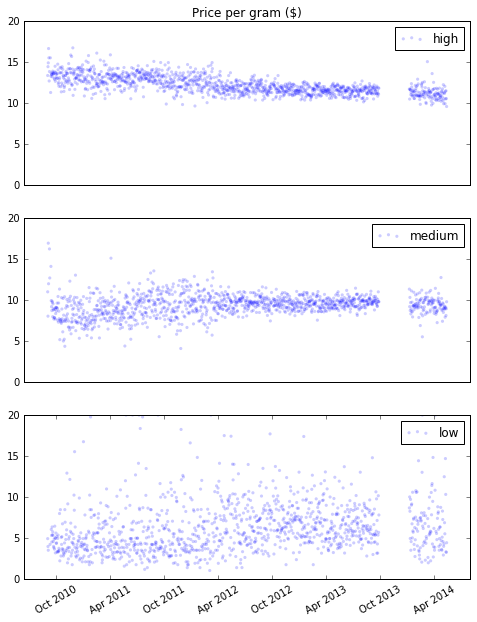We can use `statsmodels` to run a linear model of price as a function of time.

In :
```import statsmodels.formula.api as smf

def RunLinearModel(daily):
model = smf.ols('ppg ~ years', data=daily)
results = model.fit()
return model, results
```

Here's what the results look like.

In :
```from IPython.display import display

for name, daily in dailies.items():
model, results = RunLinearModel(daily)
print(name)
display(results.summary())
```
```high
```
Dep. Variable: R-squared: ppg 0.444 OLS 0.444 Least Squares 989.7 Wed, 04 Jan 2017 3.69e-160 11:44:14 -1510.1 1241 3024. 1239 3035. 1 nonrobust
coef std err t P>|t| [95.0% Conf. Int.] 13.4496 0.045 296.080 0.000 13.361 13.539 -0.7082 0.023 -31.460 0.000 -0.752 -0.664
 Omnibus: Durbin-Watson: 56.254 1.847 0 128.992 0.252 9.76e-29 4.497 4.71
```medium
```
Dep. Variable: R-squared: ppg 0.050 OLS 0.049 Least Squares 64.92 Wed, 04 Jan 2017 1.82e-15 11:44:14 -2053.9 1238 4112. 1236 4122. 1 nonrobust
coef std err t P>|t| [95.0% Conf. Int.] 8.8791 0.071 125.043 0.000 8.740 9.018 0.2832 0.035 8.057 0.000 0.214 0.352
 Omnibus: Durbin-Watson: 133.025 1.767 0 630.863 0.385 1.02e-137 6.411 4.73
```low
```
Dep. Variable: R-squared: ppg 0.030 OLS 0.029 Least Squares 35.90 Wed, 04 Jan 2017 2.76e-09 11:44:14 -3091.3 1179 6187. 1177 6197. 1 nonrobust
coef std err t P>|t| [95.0% Conf. Int.] 5.3616 0.194 27.671 0.000 4.981 5.742 0.5683 0.095 5.991 0.000 0.382 0.754
 Omnibus: Durbin-Watson: 649.338 1.82 0 6347.61 2.373 0 13.329 4.85

Now let's plot the fitted model with the data.

In :
```def PlotFittedValues(model, results, label=''):
"""Plots original data and fitted values.

model: StatsModel model object
results: StatsModel results object
"""
years = model.exog[:,1]
values = model.endog
thinkplot.Scatter(years, values, s=15, label=label)
thinkplot.Plot(years, results.fittedvalues, label='model', color='#ff7f00')
```

The following function plots the original data and the fitted curve.

In :
```def PlotLinearModel(daily, name):
"""Plots a linear fit to a sequence of prices, and the residuals.

daily: DataFrame of daily prices
name: string
"""
model, results = RunLinearModel(daily)
PlotFittedValues(model, results, label=name)
thinkplot.Config(title='Fitted values',
xlabel='Years',
xlim=[-0.1, 3.8],
ylabel='Price per gram (\$)')
```

Here are results for the high quality category:

In :
```name = 'high'
daily = dailies[name]

PlotLinearModel(daily, name)
```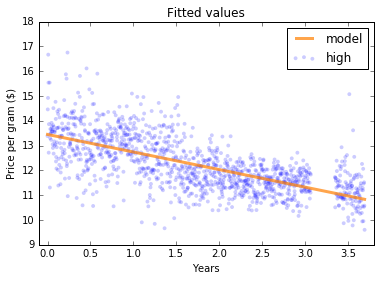## Moving averages¶

As a simple example, I'll show the rolling average of the numbers from 1 to 10.

In :
```series = np.arange(10)
```

With a "window" of size 3, we get the average of the previous 3 elements, or nan when there are fewer than 3.

In :
```pd.rolling_mean(series, 3)
```
Out:
`array([ nan,  nan,   1.,   2.,   3.,   4.,   5.,   6.,   7.,   8.])`

The following function plots the rolling mean.

In :
```def PlotRollingMean(daily, name):
"""Plots rolling mean.

daily: DataFrame of daily prices
"""
dates = pd.date_range(daily.index.min(), daily.index.max())
reindexed = daily.reindex(dates)

thinkplot.Scatter(reindexed.ppg, s=15, alpha=0.2, label=name)
roll_mean = pd.rolling_mean(reindexed.ppg, 30)
thinkplot.Plot(roll_mean, label='rolling mean', color='#ff7f00')
plt.xticks(rotation=30)
thinkplot.Config(ylabel='price per gram (\$)')
```

Here's what it looks like for the high quality category.

In :
```PlotRollingMean(daily, name)
```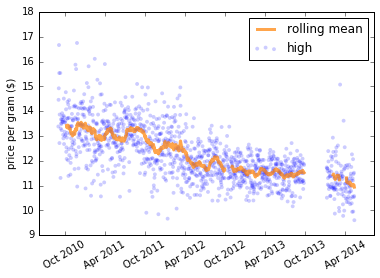The exponentially-weighted moving average gives more weight to more recent points.

In :
```def PlotEWMA(daily, name):
"""Plots rolling mean.

daily: DataFrame of daily prices
"""
dates = pd.date_range(daily.index.min(), daily.index.max())
reindexed = daily.reindex(dates)

thinkplot.Scatter(reindexed.ppg, s=15, alpha=0.2, label=name)
roll_mean = pd.ewma(reindexed.ppg, 30)
thinkplot.Plot(roll_mean, label='EWMA', color='#ff7f00')
plt.xticks(rotation=30)
thinkplot.Config(ylabel='price per gram (\$)')
```
In :
```PlotEWMA(daily, name)
```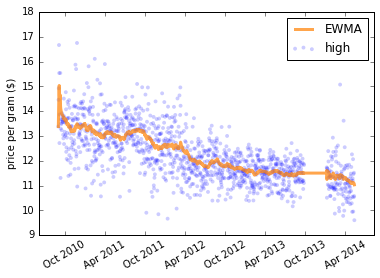We can use resampling to generate missing values with the right amount of noise.

In :
```def FillMissing(daily, span=30):
"""Fills missing values with an exponentially weighted moving average.

Resulting DataFrame has new columns 'ewma' and 'resid'.

daily: DataFrame of daily prices
span: window size (sort of) passed to ewma

returns: new DataFrame of daily prices
"""
dates = pd.date_range(daily.index.min(), daily.index.max())
reindexed = daily.reindex(dates)

ewma = pd.ewma(reindexed.ppg, span=span)

resid = (reindexed.ppg - ewma).dropna()
fake_data = ewma + thinkstats2.Resample(resid, len(reindexed))
reindexed.ppg.fillna(fake_data, inplace=True)

reindexed['ewma'] = ewma
reindexed['resid'] = reindexed.ppg - ewma
return reindexed
```
In :
```def PlotFilled(daily, name):
"""Plots the EWMA and filled data.

daily: DataFrame of daily prices
"""
filled = FillMissing(daily, span=30)
thinkplot.Scatter(filled.ppg, s=15, alpha=0.2, label=name)
thinkplot.Plot(filled.ewma, label='EWMA', color='#ff7f00')
plt.xticks(rotation=30)
thinkplot.Config(ylabel='Price per gram (\$)')
```

Here's what the EWMA model looks like with missing values filled.

In :
```PlotFilled(daily, name)
```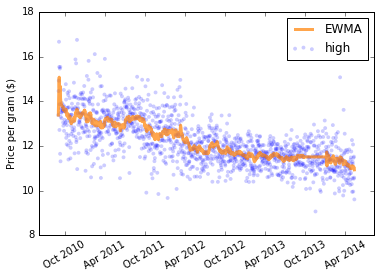## Serial correlation¶

The following function computes serial correlation with the given lag.

In :
```def SerialCorr(series, lag=1):
xs = series[lag:]
ys = series.shift(lag)[lag:]
corr = thinkstats2.Corr(xs, ys)
return corr
```

Before computing correlations, we'll fill missing values.

In :
```filled_dailies = {}
for name, daily in dailies.items():
filled_dailies[name] = FillMissing(daily, span=30)
```

Here are the serial correlations for raw price data.

In :
```for name, filled in filled_dailies.items():
corr = thinkstats2.SerialCorr(filled.ppg, lag=1)
print(name, corr)
```
```high 0.480157057617
medium 0.1736217448
low 0.119991439308
```

It's not surprising that there are correlations between consecutive days, because there are obvious trends in the data.

It is more interested to see whether there are still correlations after we subtract away the trends.

In :
```for name, filled in filled_dailies.items():
corr = thinkstats2.SerialCorr(filled.resid, lag=1)
print(name, corr)
```
```high -0.0164485711933
medium -0.0181750319436
low 0.0469291300611
```

Even if the correlations between consecutive days are weak, there might be correlations across intervals of one week, one month, or one year.

In :
```rows = []
for lag in [1, 7, 30, 365]:
print(lag, end='\t')
for name, filled in filled_dailies.items():
corr = SerialCorr(filled.resid, lag)
print('%.2g' % corr, end='\t')
print()
```
```1 -0.016 -0.018 0.047
7 0.0032 -0.032 -0.019
30 0.011 -0.0014 -0.017
365 0.051 0.013 0.026
```

The strongest correlation is a weekly cycle in the medium quality category.

## Autocorrelation¶

The autocorrelation function is the serial correlation computed for all lags.

We can use it to replicate the results from the previous section.

In :
```import statsmodels.tsa.stattools as smtsa

filled = filled_dailies['high']
acf = smtsa.acf(filled.resid, nlags=365, unbiased=True)
print('%0.2g, %.2g, %0.2g, %0.2g, %0.2g' %
(acf, acf, acf, acf, acf))
```
```1, -0.016, 0.0031, 0.011, 0.049
```

To get a sense of how much autocorrelation we should expect by chance, we can resample the data (which eliminates any actual autocorrelation) and compute the ACF.

In :
```def SimulateAutocorrelation(daily, iters=1001, nlags=40):
"""Resample residuals, compute autocorrelation, and plot percentiles.

daily: DataFrame
iters: number of simulations to run
nlags: maximum lags to compute autocorrelation
"""
# run simulations
t = []
for _ in range(iters):
filled = FillMissing(daily, span=30)
resid = thinkstats2.Resample(filled.resid)
acf = smtsa.acf(resid, nlags=nlags, unbiased=True)[1:]
t.append(np.abs(acf))

high = thinkstats2.PercentileRows(t, [97.5])
low = -high
lags = range(1, nlags+1)
thinkplot.FillBetween(lags, low, high, alpha=0.2, color='gray')
```

The following function plots the actual autocorrelation for lags up to 40 days.

The flag `add_weekly` indicates whether we should add a simulated weekly cycle.

In :
```def PlotAutoCorrelation(dailies, nlags=40, add_weekly=False):
"""Plots autocorrelation functions.

dailies: map from category name to DataFrame of daily prices
nlags: number of lags to compute
add_weekly: boolean, whether to add a simulated weekly pattern
"""
thinkplot.PrePlot(3)
daily = dailies['high']
SimulateAutocorrelation(daily)

for name, daily in dailies.items():

if add_weekly:
daily = AddWeeklySeasonality(daily)

filled = FillMissing(daily, span=30)

acf = smtsa.acf(filled.resid, nlags=nlags, unbiased=True)
lags = np.arange(len(acf))
thinkplot.Plot(lags[1:], acf[1:], label=name)
```

To show what a strong weekly cycle would look like, we have the option of adding a price increase of 1-2 dollars on Friday and Saturdays.

In :
```def AddWeeklySeasonality(daily):
"""Adds a weekly pattern.

daily: DataFrame of daily prices

returns: new DataFrame of daily prices
"""
fri_or_sat = (daily.index.dayofweek==4) | (daily.index.dayofweek==5)
fake = daily.copy()
fake.ppg.loc[fri_or_sat] += np.random.uniform(0, 2, fri_or_sat.sum())
return fake
```

Here's what the real ACFs look like. The gray regions indicate the levels we expect by chance.

In :
```axis = [0, 41, -0.2, 0.2]

PlotAutoCorrelation(dailies, add_weekly=False)
thinkplot.Config(axis=axis,
loc='lower right',
ylabel='correlation',
xlabel='lag (day)')
```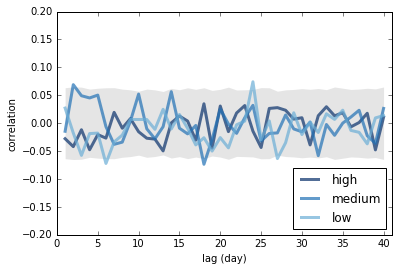Here's what it would look like if there were a weekly cycle.

In :
```PlotAutoCorrelation(dailies, add_weekly=True)
thinkplot.Config(axis=axis,
loc='lower right',
xlabel='lag (days)')
```
```/home/downey/anaconda2/lib/python2.7/site-packages/pandas/core/indexing.py:128: SettingWithCopyWarning:
A value is trying to be set on a copy of a slice from a DataFrame

See the caveats in the documentation: http://pandas.pydata.org/pandas-docs/stable/indexing.html#indexing-view-versus-copy
self._setitem_with_indexer(indexer, value)
```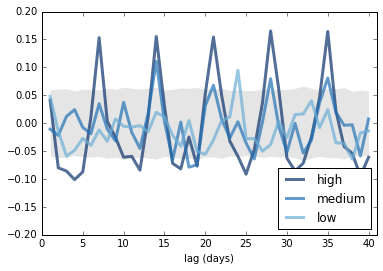## Prediction¶

The simplest way to generate predictions is to use `statsmodels` to fit a model to the data, then use the `predict` method from the results.

In :
```def GenerateSimplePrediction(results, years):
"""Generates a simple prediction.

results: results object
years: sequence of times (in years) to make predictions for

returns: sequence of predicted values
"""
n = len(years)
inter = np.ones(n)
d = dict(Intercept=inter, years=years, years2=years**2)
predict_df = pd.DataFrame(d)
predict = results.predict(predict_df)
return predict
```
In :
```def PlotSimplePrediction(results, years):
predict = GenerateSimplePrediction(results, years)

thinkplot.Scatter(daily.years, daily.ppg, alpha=0.2, label=name)
thinkplot.plot(years, predict, color='#ff7f00')
xlim = years-0.1, years[-1]+0.1
thinkplot.Config(title='Predictions',
xlabel='Years',
xlim=xlim,
ylabel='Price per gram (\$)',
loc='upper right')
```

Here's what the prediction looks like for the high quality category, using the linear model.

In :
```name = 'high'
daily = dailies[name]

_, results = RunLinearModel(daily)
years = np.linspace(0, 5, 101)
PlotSimplePrediction(results, years)
```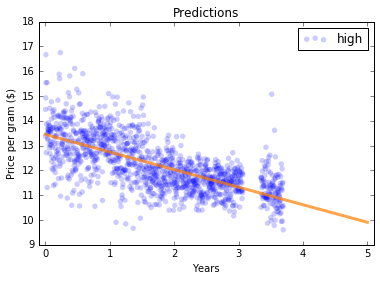When we generate predictions, we want to quatify the uncertainty in the prediction. We can do that by resampling. The following function fits a model to the data, computes residuals, then resamples from the residuals to general fake datasets. It fits the same model to each fake dataset and returns a list of results.

In :
```def SimulateResults(daily, iters=101, func=RunLinearModel):
"""Run simulations based on resampling residuals.

daily: DataFrame of daily prices
iters: number of simulations
func: function that fits a model to the data

returns: list of result objects
"""
_, results = func(daily)
fake = daily.copy()

result_seq = []
for _ in range(iters):
fake.ppg = results.fittedvalues + thinkstats2.Resample(results.resid)
_, fake_results = func(fake)
result_seq.append(fake_results)

return result_seq
```

To generate predictions, we take the list of results fitted to resampled data. For each model, we use the `predict` method to generate predictions, and return a sequence of predictions.

If `add_resid` is true, we add resampled residuals to the predicted values, which generates predictions that include predictive uncertainty (due to random noise) as well as modeling uncertainty (due to random sampling).

In :
```def GeneratePredictions(result_seq, years, add_resid=False):
"""Generates an array of predicted values from a list of model results.

When add_resid is False, predictions represent sampling error only.

When add_resid is True, they also include residual error (which is
more relevant to prediction).

result_seq: list of model results
years: sequence of times (in years) to make predictions for
add_resid: boolean, whether to add in resampled residuals

returns: sequence of predictions
"""
n = len(years)
d = dict(Intercept=np.ones(n), years=years, years2=years**2)
predict_df = pd.DataFrame(d)

predict_seq = []
for fake_results in result_seq:
predict = fake_results.predict(predict_df)
if add_resid:
predict += thinkstats2.Resample(fake_results.resid, n)
predict_seq.append(predict)

return predict_seq
```

To visualize predictions, I show a darker region that quantifies modeling uncertainty and a lighter region that quantifies predictive uncertainty.

In :
```def PlotPredictions(daily, years, iters=101, percent=90, func=RunLinearModel):
"""Plots predictions.

daily: DataFrame of daily prices
years: sequence of times (in years) to make predictions for
iters: number of simulations
percent: what percentile range to show
func: function that fits a model to the data
"""
result_seq = SimulateResults(daily, iters=iters, func=func)
p = (100 - percent) / 2
percents = p, 100-p

predict_seq = GeneratePredictions(result_seq, years, add_resid=True)
low, high = thinkstats2.PercentileRows(predict_seq, percents)
thinkplot.FillBetween(years, low, high, alpha=0.3, color='gray')

predict_seq = GeneratePredictions(result_seq, years, add_resid=False)
low, high = thinkstats2.PercentileRows(predict_seq, percents)
thinkplot.FillBetween(years, low, high, alpha=0.5, color='gray')
```

Here are the results for the high quality category.

In :
```years = np.linspace(0, 5, 101)
thinkplot.Scatter(daily.years, daily.ppg, alpha=0.1, label=name)
PlotPredictions(daily, years)
xlim = years-0.1, years[-1]+0.1
thinkplot.Config(title='Predictions',
xlabel='Years',
xlim=xlim,
ylabel='Price per gram (\$)')
```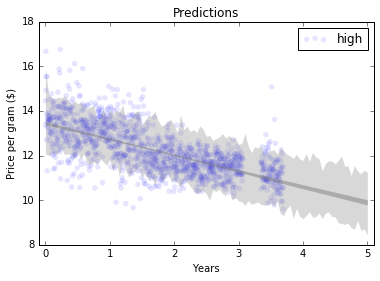But there is one more source of uncertainty: how much past data should we use to build the model?

The following function generates a sequence of models based on different amounts of past data.

In :
```def SimulateIntervals(daily, iters=101, func=RunLinearModel):
"""Run simulations based on different subsets of the data.

daily: DataFrame of daily prices
iters: number of simulations
func: function that fits a model to the data

returns: list of result objects
"""
result_seq = []
starts = np.linspace(0, len(daily), iters).astype(int)

for start in starts[:-2]:
subset = daily[start:]
_, results = func(subset)
fake = subset.copy()

for _ in range(iters):
fake.ppg = (results.fittedvalues +
thinkstats2.Resample(results.resid))
_, fake_results = func(fake)
result_seq.append(fake_results)

return result_seq
```

And this function plots the results.

In :
```def PlotIntervals(daily, years, iters=101, percent=90, func=RunLinearModel):
"""Plots predictions based on different intervals.

daily: DataFrame of daily prices
years: sequence of times (in years) to make predictions for
iters: number of simulations
percent: what percentile range to show
func: function that fits a model to the data
"""
result_seq = SimulateIntervals(daily, iters=iters, func=func)
p = (100 - percent) / 2
percents = p, 100-p

predict_seq = GeneratePredictions(result_seq, years, add_resid=True)
low, high = thinkstats2.PercentileRows(predict_seq, percents)
thinkplot.FillBetween(years, low, high, alpha=0.2, color='gray')
```

Here's what the high quality category looks like if we take into account uncertainty about how much past data to use.

In :
```name = 'high'
daily = dailies[name]

thinkplot.Scatter(daily.years, daily.ppg, alpha=0.1, label=name)
PlotIntervals(daily, years)
PlotPredictions(daily, years)
xlim = years-0.1, years[-1]+0.1
thinkplot.Config(title='Predictions',
xlabel='Years',
xlim=xlim,
ylabel='Price per gram (\$)')
```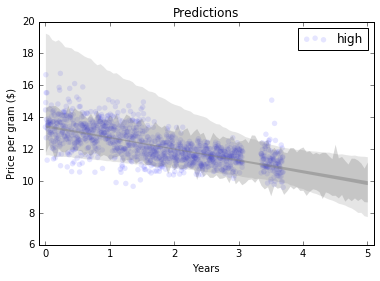## Exercises¶

Exercise: The linear model I used in this chapter has the obvious drawback that it is linear, and there is no reason to expect prices to change linearly over time. We can add flexibility to the model by adding a quadratic term, as we did in Section 11.3.

Use a quadratic model to fit the time series of daily prices, and use the model to generate predictions. You will have to write a version of `RunLinearModel` that runs that quadratic model, but after that you should be able to reuse code from the chapter to generate predictions.

In :
```# Solution

def RunQuadraticModel(daily):
"""Runs a linear model of prices versus years.

daily: DataFrame of daily prices

returns: model, results
"""
daily['years2'] = daily.years**2
model = smf.ols('ppg ~ years + years2', data=daily)
results = model.fit()
return model, results
```
In :
```# Solution

name = 'high'
daily = dailies[name]

model, results = RunQuadraticModel(daily)
results.summary()
```
Out:
Dep. Variable: R-squared: ppg 0.455 OLS 0.454 Least Squares 517.5 Wed, 04 Jan 2017 4.57e-164 11:45:26 -1497.4 1241 3001. 1238 3016. 2 nonrobust
coef std err t P>|t| [95.0% Conf. Int.] 13.6980 0.067 205.757 0.000 13.567 13.829 -1.1171 0.084 -13.326 0.000 -1.282 -0.953 0.1132 0.022 5.060 0.000 0.069 0.157
 Omnibus: Durbin-Watson: 49.112 1.885 0 113.885 0.199 1.86e-25 4.43 27.5
In :
```# Solution

PlotFittedValues(model, results, label=name)
thinkplot.Config(title='Fitted values',
xlabel='Years',
xlim=[-0.1, 3.8],
ylabel='price per gram (\$)')
```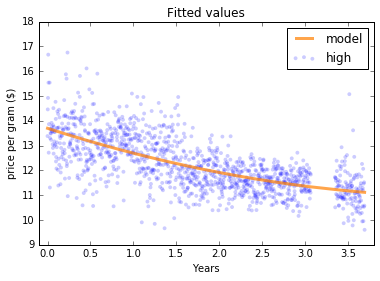In :
```# Solution

years = np.linspace(0, 5, 101)
thinkplot.Scatter(daily.years, daily.ppg, alpha=0.1, label=name)
PlotPredictions(daily, years, func=RunQuadraticModel)
thinkplot.Config(title='predictions',
xlabel='Years',
xlim=[years-0.1, years[-1]+0.1],
ylabel='Price per gram (\$)')
```